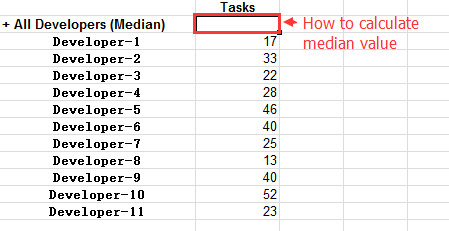I want to calculate the median value, how to write this formula,thank you!Hi,

To calculate the median value of all children of the expanded group-member (All Developers) you could try using the “Median” function with “Descendants”.

``````Median(
Filter(
Descendants([Developer].CurrentMember, [Developer].[Developer]),